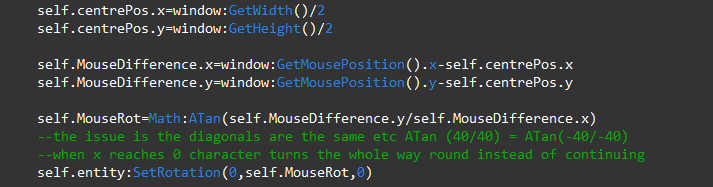## Recommended Posts

so I try to get the center of the screen and find the mouse position and then find the difference then using ATan(change in y/ change in x) to find the angle the object must rotate to and set the angle to that, the only issue is when the mouse x is <= 0 the object flips (so lets say the right side of the object was facing the mouse it then becomes the left side) I assume this issue is to do with the fact that ATan(change in y/change in x)=ATan(-change in y/-change in x) but here is my code:So you are only setting the y rotation of lets say your character controller.
Why would you not just rotate it based on direction vector and make it really small ?

local rotVec = window:GetMousePosition()x-(window:GetWidth*0.5)

local lookSpeedMultiplier = 0.01

self.entity:GetRotation(0, self.entity.rotation.y + (rotVec * lookSpeedMultiplier), 0)

I just wrote the code in here so there might be errors but it may work. Also always make *0.5 instead of /2 because the compiler could not detect that /2 is more difficult to calculate than *0.5

12 hours ago, Slastraf said:

So you are only setting the y rotation﻿ of lets say your character controller.
Why would you not just rotate it based on direction vector a﻿nd make it really small ?

local rotVec = window:GetMousePosition()x-(window:GetWidth*0.5)

local lookSpeedMultiplier = 0.01

self.entity:GetRotation(0, self.entity.rotation.y + (rotVec * lookSpeedMultiplier), 0) ﻿﻿﻿﻿﻿﻿

I just wrote the code in here so there might be errors but it may work. Also always make *0.5 instead of /2 because the compiler could not detect that /2 is more ﻿difficult to calculate than *0.5

I don't think this is the right thing because I want it to rotate towards the mouse yet this will rotate it constantly however, there is some good use in this as if I make a rotVec for the y coordinates as well and do the whole Rot=ATan(rotVecy/rotVecx) thing and then do self.entity:SetRotation(0,self.entity:GetRotation().y+(Rot*lookSpeedMultiplier),0) and then run the code you can see that the moment you cross where x=0 the entity starts rotating the other way and I assume this is the base of the issue.

4 hours ago, hom.png said:

I don't think this is the right thing because I want it to rotate towards the mouse yet this will rotate it constantly however, there is some good use in this as if I make a rotVec for the y coordinates as well and do the whole Rot=ATan(rotVecy/rotVecx) thing and then do self.entity:SetRotation(0,self.entity:GetRotation().y+(Rot*lookSpeedMultiplier),0) and then run the code you can see that the moment you cross where x=0 the entity starts rotating the other way and I assume this is the base of the issue.

you would need to put the mouse back, maybe use Math Lerp function for that.

## Join the conversation

You can post now and register later. If you have an account, sign in now to post with your account.×   Pasted as rich text.   Paste as plain text instead

Only 75 emoji are allowed.

×   Your previous content has been restored.   Clear editor

×   You cannot paste images directly. Upload or insert images from URL.

××

• Pages

• Back
• Store

• #### Support

• Projects
• Gallery
×
• Create New...